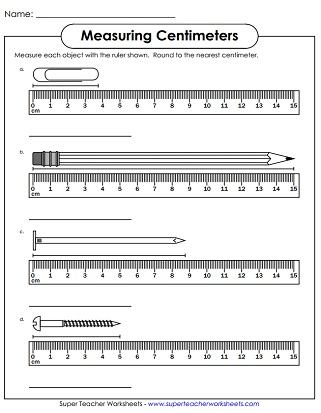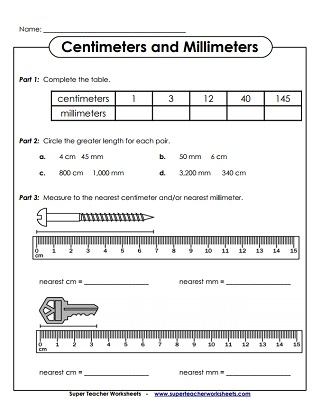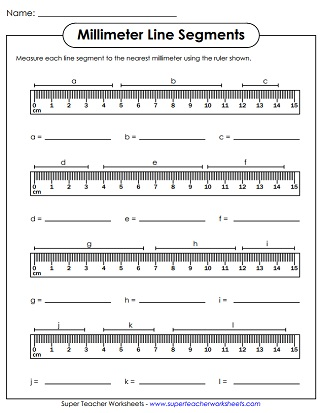# Metric Measurement Worksheets: cm, mm, km, and m

Metric linear measurement worksheets for measuring and converting centimeters, millimeters, kilometers, and meters.## Measuring Centimeters

Measure each object to the nearest centimeter using the ruler shown
Measure each of the objects to the nearest cm using the shown ruler
Use this worksheet to help your students practice using a ruler and measuring to the nearest centimeter.
Use this worksheet to have students practice measuring to the nearest centimeter.
With these flash cards, students will be able to practice measuring objects to the nearest centimeter.
Write the approximate measurement of each, rounding to the nearest centimeter. Then tell how much longer the nail is than the paperclip. And tell how much longer the pencil is than the nail.

## Measuring Millimeters

Measure the length of each object to the nearest millimeter using the given ruler.
Measure the length of each of these objects to the nearest mm using the ruler illustration.

Use these task cards with your class to help them practice converting between meters and kilometers.
Use these task cards to help students practice converting between centimeters, millimeters, and meters.
Use these task cards to help students practice converting millimeters, centimeters, meters, and kilometers.

## Mixed-Unit Measuring and Converting

Practice measuring with centimeters and millimeters with this worksheet.
With this worksheet, students will practice using a ruler and measuring to the nearest centimeter and millimeter.
Have your students cut out these flash cards and use them to practice measuring objects to the nearest centimeter and millimeter.
Measure centimeters and millimeters, convert from centimeters to millimeters, circle the greater length, and more.  2-sided sheet could be a review or a quiz.
In-and-out boxes for converting mm, cm, m, and km; includes word problems.
Use this worksheet to help students become familiar with converting metric linear units.
This worksheet includes two word problems in addition to the basic conversion questions.
Practice converting linear metric measurements. Includes decimals.
These basic conversions include only whole numbers and the units millimeter, centimeter, and meter.
This conversion worksheet includes decimals and two multi-step word problems.
The conversion problems on this worksheet include only whole numbers.
Measurement (Standard)

Measure the length of each object to the nearest millimeter using the given ruler.

Measurement - Milliliters and Liters

Measure liquids to the nearest liter and milliliter.

Measurement - Temperature

Use the thermometer pictures to determine the temperature.

## Worksheet ImagesMy Account
Site Information